Ex 6.2

Chapter 6 Class 6 Integers
Serial order wise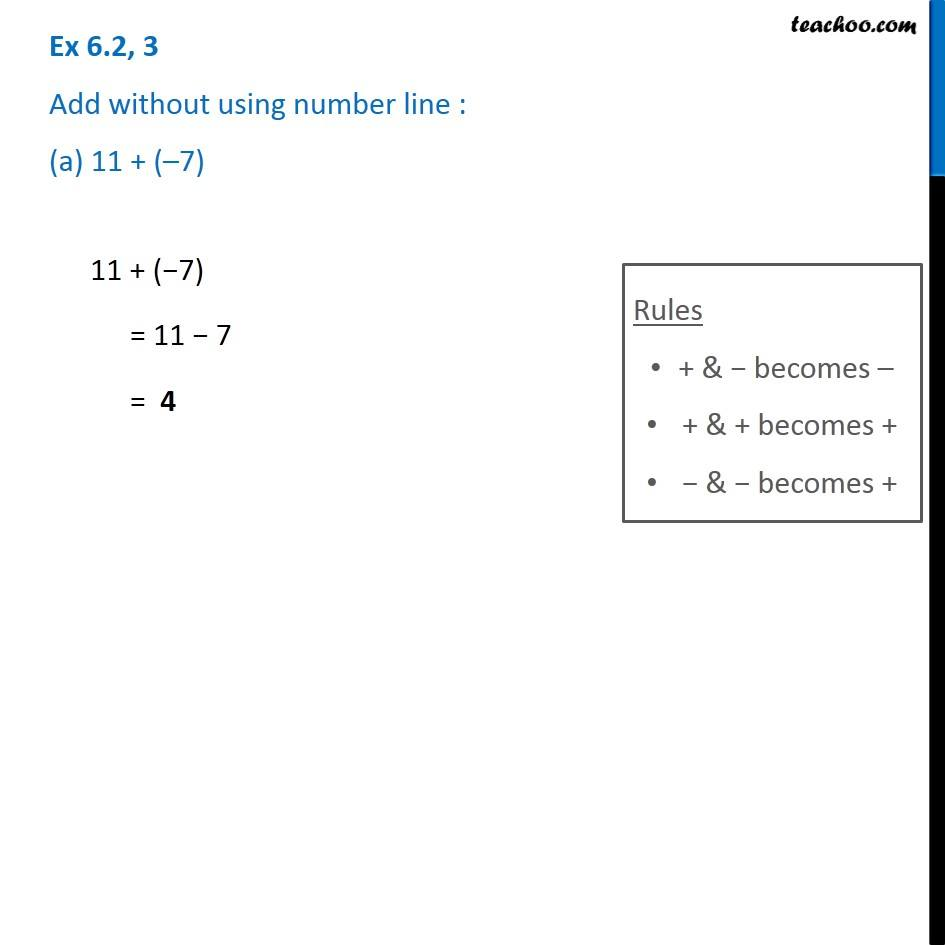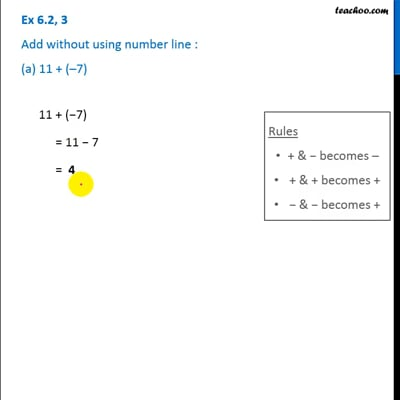This video is only available for Teachoo black users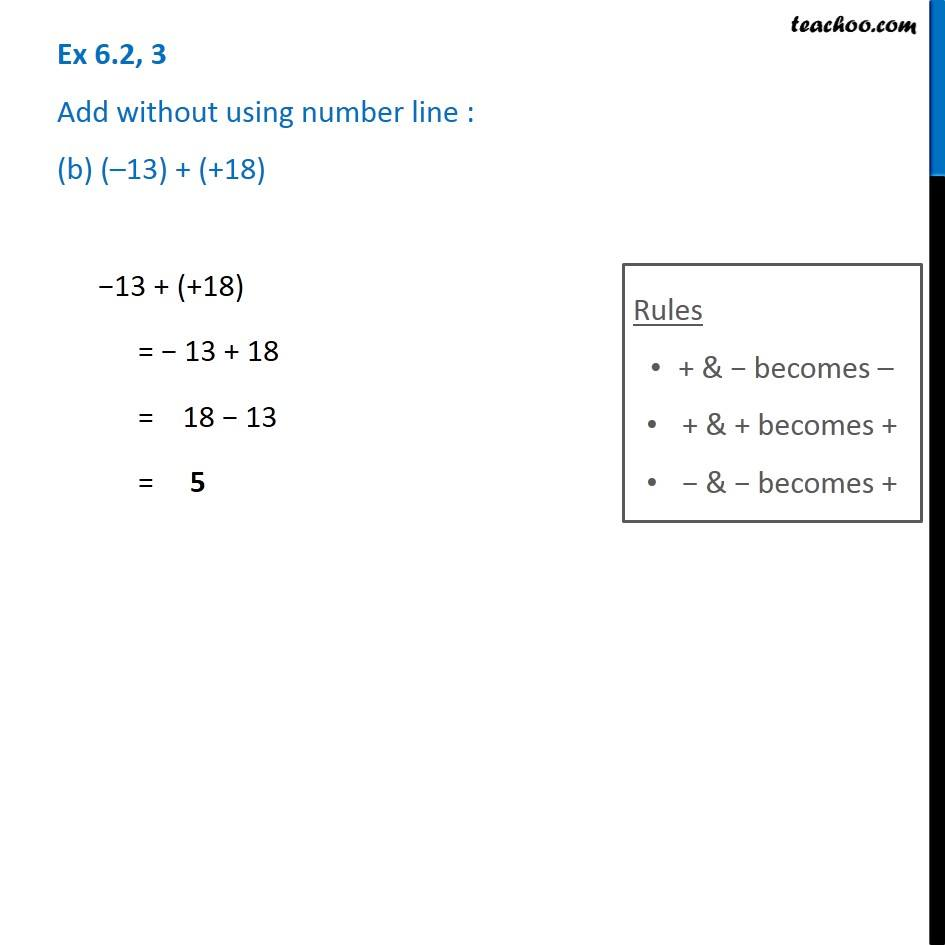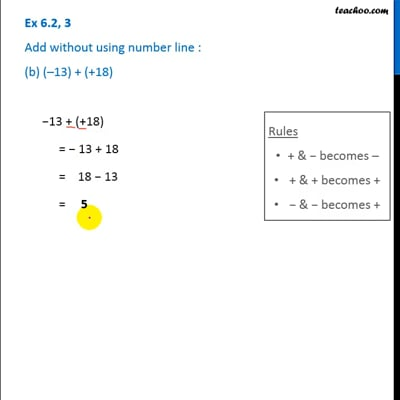This video is only available for Teachoo black users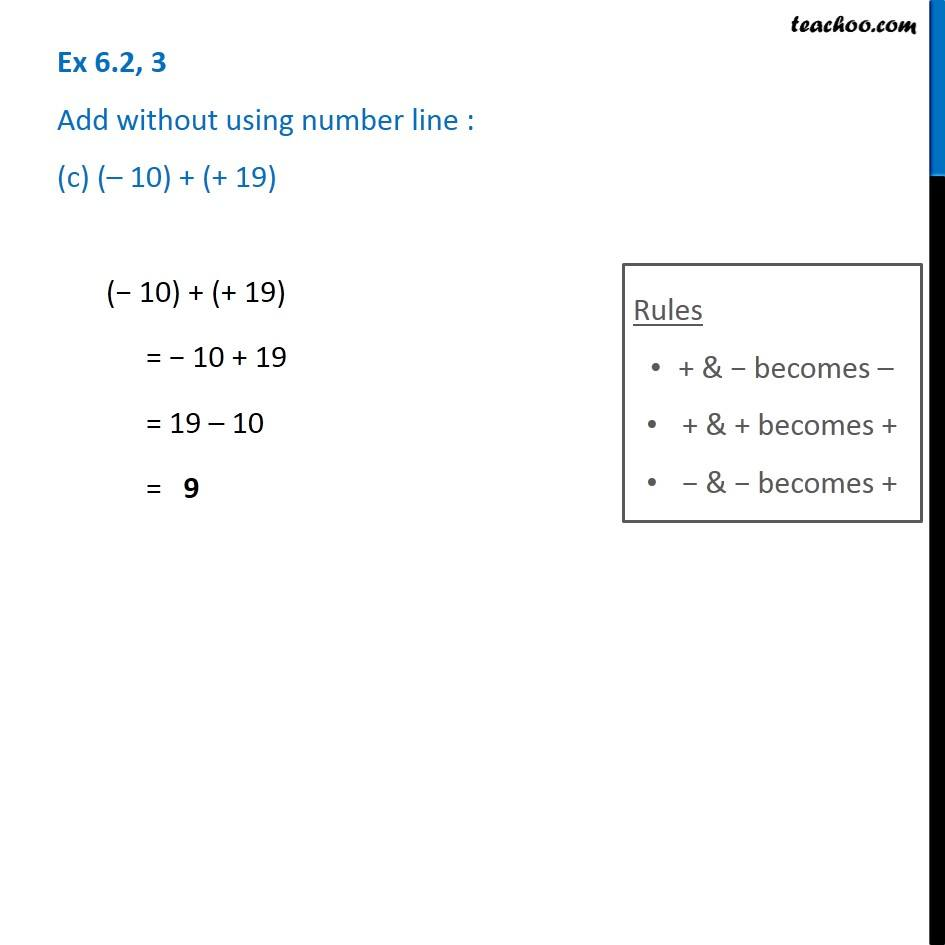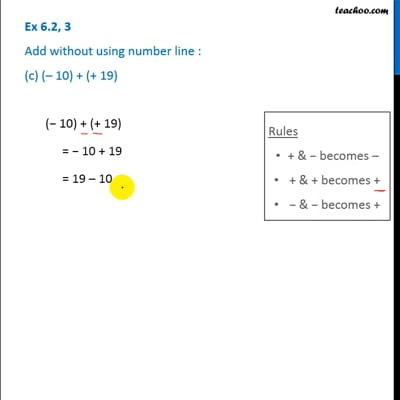This video is only available for Teachoo black users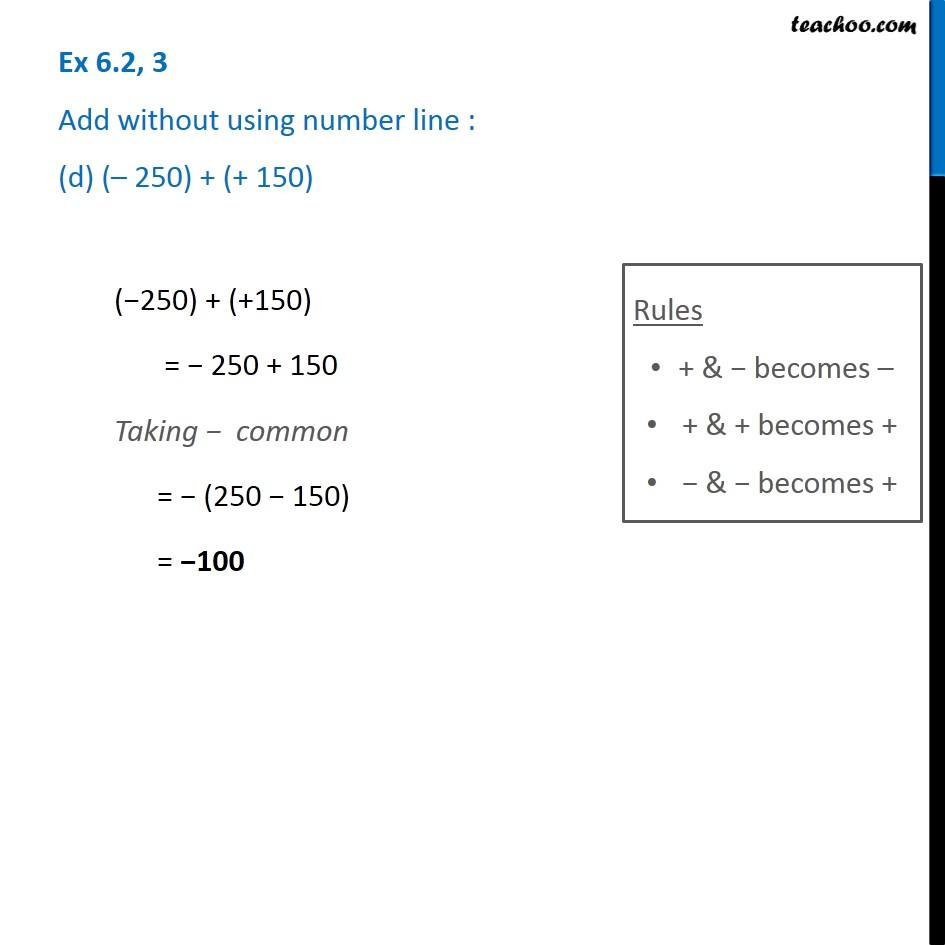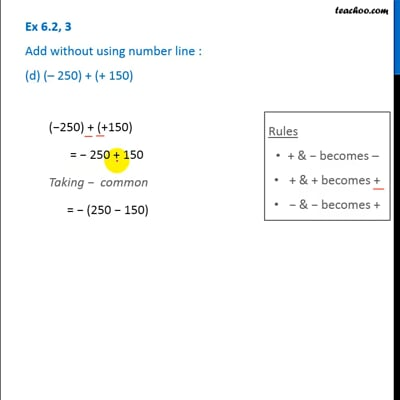This video is only available for Teachoo black users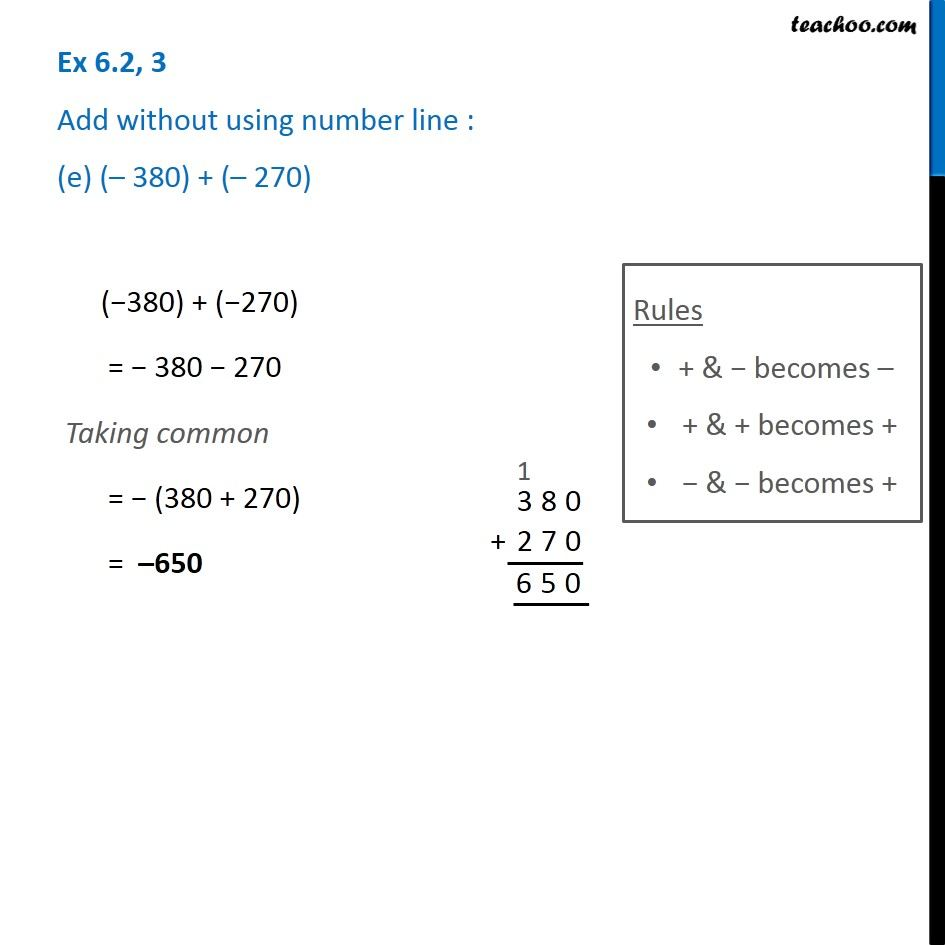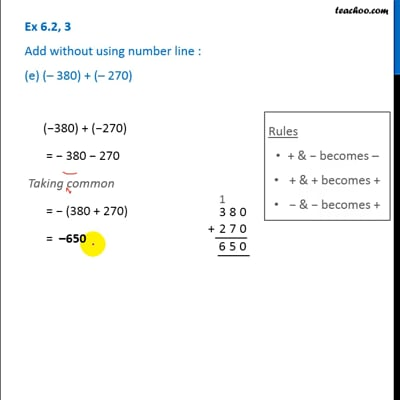This video is only available for Teachoo black users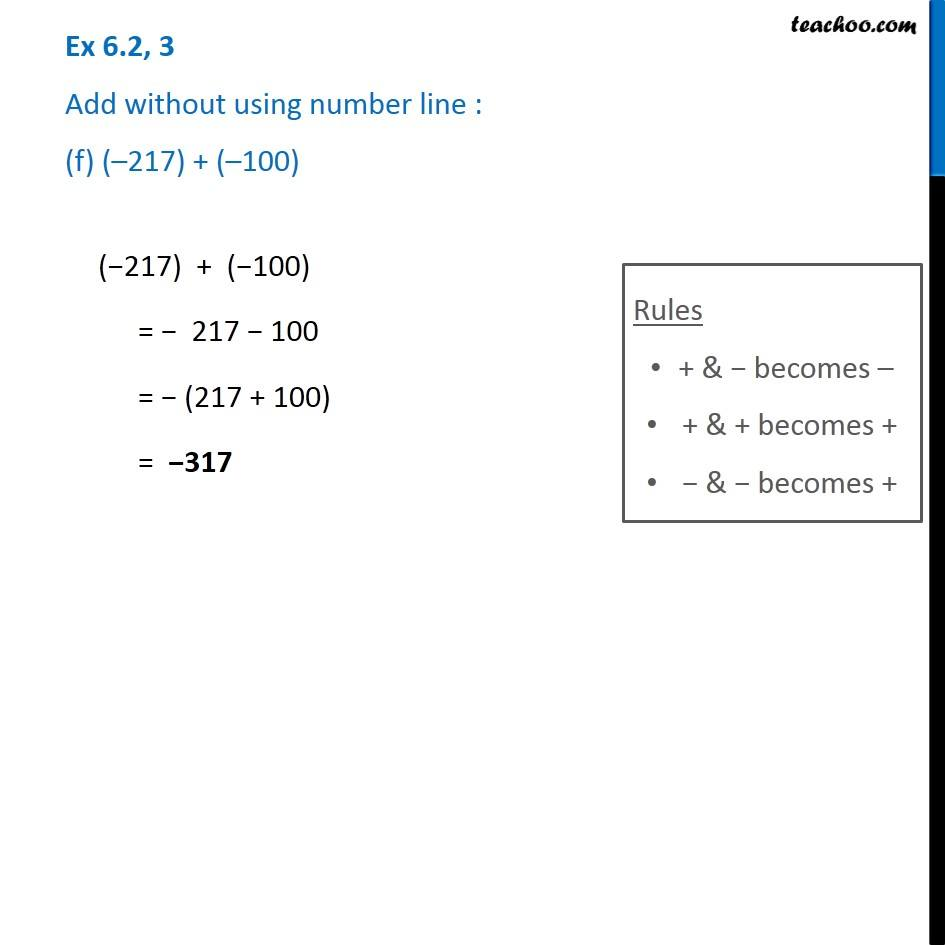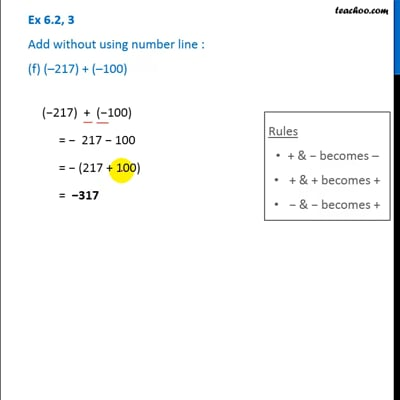This video is only available for Teachoo black users

### Transcript

Ex 6.2, 3 Add without using number line : (a) 11 + ( 7) 11 + ( 7) = 11 7 = 4 Rules + & becomes + & + becomes + & becomes + Ex 6.2, 3 Add without using number line : (b) ( 13) + (+18) 13 + (+18) = 13 + 18 = 18 13 = 5 Rules + & becomes + & + becomes + & becomes + Ex 6.2, 3 Add without using number line : (c) ( 10) + (+ 19)( 10) + (+ 19) = 10 + 19 = 19 10 = 9 Rules + & becomes + & + becomes + & becomes + Ex 6.2, 3 Add without using number line : (d) ( 250) + (+ 150)( 250) + (+150) = 250 + 150 Taking common = (250 150) = 100 Rules + & becomes + & + becomes + & becomes + Ex 6.2, 3 Add without using number line : (e) ( 380) + ( 270) ( 380) + ( 270) = 380 270 Taking common = (380 + 270) = 650 Rules + & becomes + & + becomes + & becomes + Ex 6.2, 3 Add without using number line : (f) ( 217) + ( 100)( 217) + ( 100) = 217 100 = (217 + 100) = 317